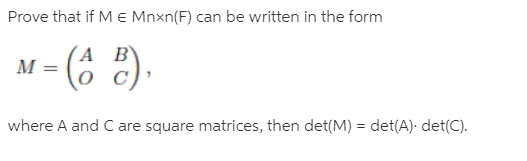# Prove that if M e Mnxn(F) can be written in the formM = (6 2).(A B'%3Dwhere A and C are square matrices, then det(M) = det(A). det(C).

Question
1 viewshelp_outlineImage TranscriptioncloseProve that if M e Mnxn(F) can be written in the form M = (6 2). (A B' %3D where A and C are square matrices, then det(M) = det(A). det(C). fullscreen

### This question hasn't been answered yet.